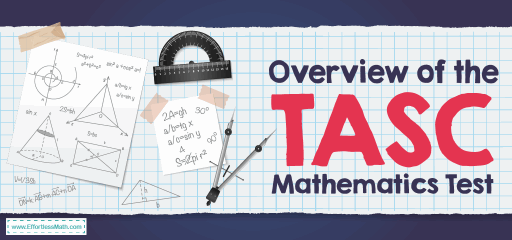# Overview of the TASC Mathematics TestTest Assessing Secondary Completion (TASC) is a test that measures readiness for the workforce or college. When a student passes the TASC, the Education Department issues them with a High School Equivalency diploma. The TASC test assesses five subject areas includes: Reading, Writing, Social Studies, Mathematics, and Science.

The purpose of the TASC math part is not only to assess your ability to solve mathematical equations but also your ability with Mathematical Reasoning. This means understanding mathematics at several levels and in several different subjects and applications. As a high school equivalency exam, the TASC is not simply an academic tool, but a tool for judging your abilities by equating principles learned academically and subjects to scenarios you could easily encounter in the real world.

The TASC test is generally available in both online or paper format and is also available in Spanish or English. There are two math parts on the TASC, spanning 42 questions if you choose to take the digital format, and 43 for the paper format. The digital format of the TASC test also includes a question in the “technology response” group. Also, you must answer one question under the “constructed response” group and 11 under the “gridded answer” subgroup. Besides, the TASC math section is split in half, for which you will receive a different duration.

Like most math tests you will face, you will be allowed to use a calculator if you need to. However, a calculator is only allowed in the first half of the math section. Before deciding which calculator to bring, make sure which models are approved, not every calculator is suitable or allowed for the test. As far as your time limit, the first half of the test should last 55 minutes (in Spanish) or 50 minutes (in English). The second half should take a full hour (in Spanish) or 55 minutes (in English), in which case you will not have access to the calculator in any form.

## What does the TASC math test cover?

The TASC mathematic questions focus on three areas:

### 1- Geometry:

Geometry includes assessing shapes on a two- and three-dimensional basis. Several questions in this knowledge group ask you to measure these shapes in specific ways – such as finding diameter, area, or perimeter. Be sure to check out the basic formulas found in geometric problems. You will want to familiarize yourself with the procedure for finding measurements detailed above and, potentially, the Pythagorean theorem, which is related to the sides and angles of triangles.

### 2- Algebra:

There are two main factors in Algebra: variables and equations. Both of these elements are closely related, so to understand one of them, you need to understand the other elements just as accurately.

### 3- Arithmetic:

Arithmetic consists of basic mathematical functions: division, multiplication, subtraction, and addition. These play an important part in higher-level mathematical subjects.

## Can you use a calculator on the TASC math test?

Texas Instrument Model TI-30XS is the preferred calculator for the TASC test. The TI-30XS is a scientific calculator with trigonometric and logarithm functions but without graph functions. Graphing calculators are not permitted for use in the TASC test. Other scientific calculators may be approved but must have trigonometric and logarithmic functions, but cannot have a graph or any programming functions.

## Do you get a formula sheet on the TASC math test?

Are you preparing for the TASC math test? First and foremost, you need to understand that the TASC® math test contains a formula sheet, which shows formulas for geometric measurements and some concepts of algebra. The formulas are provided to test-takers so that they focus more on application rather than memorizing them. However, the test does not provide a list of all the basic formulas needed to know the test. This means that you should be able to memorize many mathematical formulas in TASC.

## Is the TASC math test hard?

The TASC exam is a little more difficult than the GED exam. The TASC Math test can be challenging, but with enough practice, you can pass it successfully.

## What are the pass scores for the TASC math test?

The minimum passing score is 500 for math, and the highest possible score in this subtest is 800. If you get a high score in math and other sub-test will receive a Distinguished Achievement notation for that score. The Distinguished Achievement score is 560 for math.

## More from Effortless Math for TASC Test …

### Are you searching for a free and comprehensive TASC math practice test?

Use our free TASC Math practice tests and study resources (updated for 2022) to ace the TASC Math test!

### Are you worried that by looking at the TASC test sheet, you will forget everything you know?

Join us to learn the Top 10 Tips to Overcome TASC Math Anxiety to achieve the best result on the TASC test.

Looking for more information about TASC Testing accommodation and support for disabilities? Here is some information about special tools, materials, and accommodations that will be provided for students that need them.

## Have any questions about the TASC Test?

### What people say about "Overview of the TASC Mathematics Test - Effortless Math: We Help Students Learn to LOVE Mathematics"?

No one replied yet.

X
52% OFF

Limited time only!

Save Over 52%

SAVE \$40

It was \$76.99 now it is \$36.99